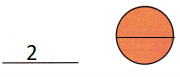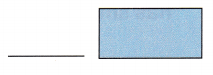# McGraw Hill Math Grade 1 Chapter 11 Lesson 12 Answer Key Making Equal Parts

All the solutions provided in McGraw Hill Math Grade 1 Answer Key PDF Chapter 11 Lesson 12 Making Equal Parts as per the latest syllabus guidelines.

## McGraw-Hill Math Grade 1 Answer Key Chapter 11 Lesson 12 Making Equal Parts

Identify

Question 1.
How many equal parts does the shape have? Is the shape divided into halves or fourths?4 equal parts.
Explanation:
The circle is divided into fourths.
the axis or imaginary line that passes through the center of the shape or object and divides it into identical halves

Question 2.
Divide the circle into halves. How many equal parts does the circle have?Explanation:
2 equal parts that the circle have.
the axis or imaginary line that passes through the center of the shape or object and divides it into identical halves

Question 3.
Divide the rectangle into fourths. How many equal parts does the rectangle have?